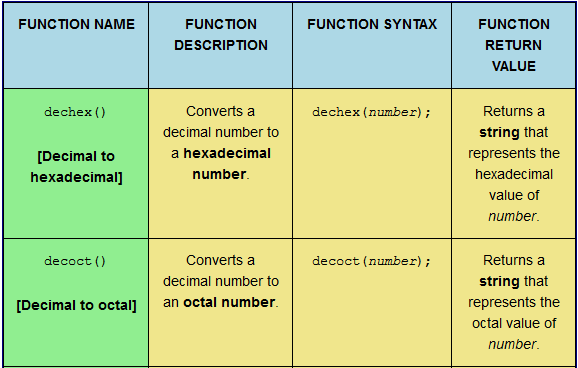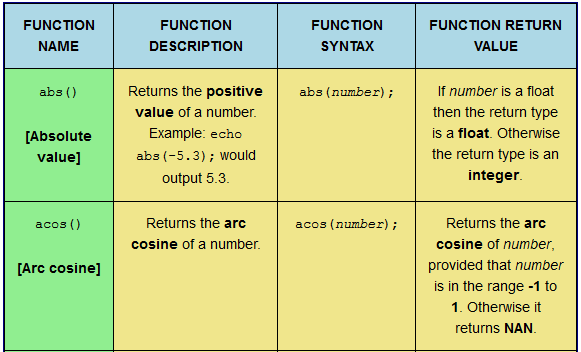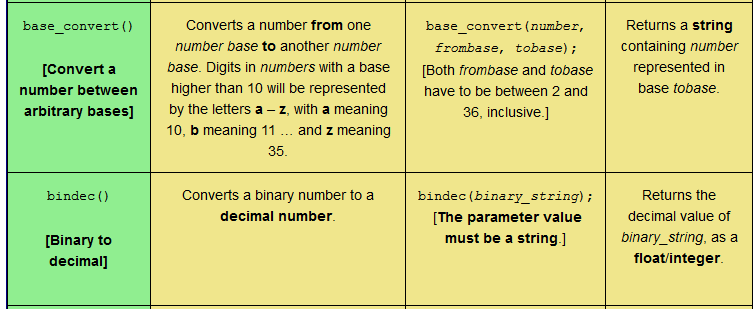PHP Mathematical Function Quiz: round(); sin(); sinh(); sqrt(); srand(); tan(); and tanh().This web page provides a reference table and a subsequent quiz to aid learning seven PHP mathematical functions: round(); sin(); sinh(); sqrt(); srand(); tan(); and tanh(). The quiz involves matching the function description, function syntax, and function return value to the correct function name. Different table formats are available in order to cater for users on a desktop computer, a laptop, or a mobile phone.

Tagged with: , , , ,
Posted in PHP Maths Quizzes

PHP Mathematical Function Quiz: mt_rand(); mt_srand(); octdec(); pi(); pow(); rad2deg(); and rand().This web page provides a reference table and a subsequent quiz to aid learning seven PHP mathematical functions: mt_rand(); mt_srand(); octdec(); pi(); pow(); rad2deg(); and rand(). The quiz involves matching the function description, function syntax, and function return value to the correct function name. Different table formats are available in order to cater for users on a desktop computer, a laptop, or a mobile phone.

Tagged with: , , , ,
Posted in PHP Maths Quizzes

PHP Mathematical Function Quiz: lcg_value(); log10(); log1p(); log(); max(); min(); and mt_getrandmax().This web page provides a reference table and a subsequent quiz to aid learning seven PHP mathematical functions: lcg_value(); log10(); log1p(); log(); max(); min(); and mt_getrandmax(). The quiz involves matching the function description, function syntax, and function return value to the correct function name. Different table formats are available in order to cater for users on a desktop computer, a laptop, or a mobile phone.

Tagged with: , , , ,
Posted in PHP Maths Quizzes

PHP Mathematical Function Quiz: getrandmax(); hexdec(); hypot(); intdiv(); is_finite(); is_infinite(); and is_nan().This web page provides a reference table and a subsequent quiz to aid learning seven PHP mathematical functions: getrandmax(); hexdec(); hypot(); intdiv(); is_finite(); is_infinite(); and is_nan(). The quiz involves matching the function description, function syntax, and function return value to the correct function name. Different table formats are available in order to cater for users on a desktop computer, a laptop, or a mobile phone.

Tagged with: , , , ,
Posted in PHP Maths Quizzes

PHP Mathematical Function Quiz: dechex(); decoct(); deg2rad(); exp(); expm1(); floor(); and fmod().This web page provides a reference table and a subsequent quiz to aid learning seven PHP mathematical functions: dechex(); decoct(); deg2rad(); exp(); expm1(); floor(); and fmod(). The quiz involves matching the function description, function syntax, and function return value to the correct function name. Different table formats are available in order to cater for users on a desktop computer, a laptop, or a mobile phone.

Tagged with: , , , ,
Posted in PHP Maths Quizzes

PHP Mathematical Function Quiz: atanh(); base_convert(); bindec(); ceil(); cos(); cosh(); and decbin().This web page provides a reference table and a subsequent quiz to aid learning seven PHP mathematical functions: atanh(); base_convert(); bindec(); ceil(); cos(); cosh(); and decbin(). The quiz involves matching the function description, function syntax, and function return value to the correct function name. Different table formats are available in order to cater for users on a desktop computer, a laptop, or a mobile phone.

Tagged with: , , , ,
Posted in PHP Maths Quizzes

PHP Mathematical Function Quiz: abs(); acos(); acosh(); asin(); asinh(); atan(); and atan2().This web page provides a reference table and a subsequent quiz to aid learning seven PHP mathematical functions: abs(); acos(); acosh(); asin(); asinh(); atan(); and atan2(). The quiz involves matching the function description, function syntax, and function return value to the correct function name. Different table formats are available in order to cater for users on a desktop computer, a laptop, or a mobile phone.

Tagged with: , , ,
Posted in PHP Maths Quizzes

PHP QUIZ: All Mathematical FunctionsThis web page provides a reference table and a subsequent quiz to aid learning forty-nine PHP mathematical functions. The quiz involves matching the function description, function syntax, and function return value to the correct function name. Different table formats are available in order to cater for users on a desktop computer, a laptop, or a mobile phone.

Tagged with: , , , ,
Posted in PHP Maths Quizzes